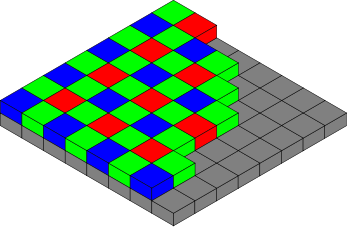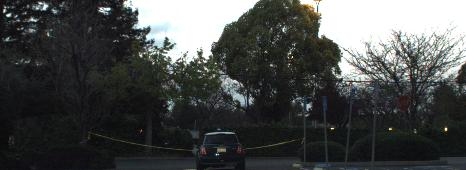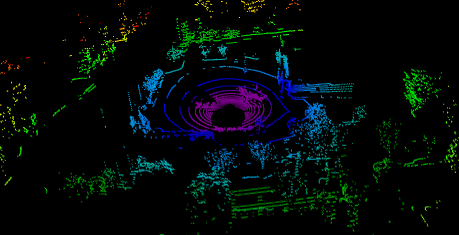## ROS and ROS bags - Part3

March 31, 2017, 9:20 p.m.

## Summary

Today I continued with extracting data from the rosbag files. I made improvements on extracting the images, and also worked on extracting the lidar point clouds.

# Color images

In yesterdays blog i extracted the images, but the images didnt seem to contain any color data. Actually it turns out I was wrong. The images do contain color data, except that it is not stored in separate channels. The images are encoded in a bayer color format which encodes different colors in adjacent pixel positions, in a grid like pattern as shown in the following image (courtesy of user Cburnett on wikipedia)This can be converted to an RGB image using openCV.

```from PIL import Image
import cv2
img = np.fromstring(msg.data, np.uint8)
img = img.reshape(msg.height, msg.width)
img = cv2.cvtColor(img, cv2.COLOR_BayerGR2BGR)
Image.fromarray(img).show()
```A video of all the frames from one bag extracted in this way is below.

## Updated Code for Processing all Images in Bag

Now its time to update the code for processing all the images from a rosbag so that color is encoded in RGB.

```import numpy as np
import cv2
import rosbag
bag = rosbag.Bag(bag_file, "r")
num_images = bag.get_message_count(topic_filters=["/image_raw"])

for i in range(num_images):
# READ NEXT MESSAGE IN BAG
topic, msg, t  = messages.next()

# CONVERT MESSAGE TO A NUMPY ARRAY
img = np.fromstring(msg.data, dtype=np.uint8)
img = img.reshape(msg.height, msg.width)

# CONVERT TO RGB
img = cv2.cvtColor(img, cv2.COLOR_BayerGR2BGR)

# DO SOME PROCESSING ON THE IMAGE
# ...
```

## Lidar Point Clouds

I tried a similar apporach for extracting the lidar data, by using:

```lidar = np.fromstring(msg.data, dtype=np.float32)
lidar = lidar.reshape(-1,4)
```

Which seemed ok, but there were some strange things about the values, the ranges of values just didnt quite seem right, and when i tried to visualize the data using mayavi, I didnt get anything recognizable in the scene. So i decided to have a search on google to find something that might be useful.

I stumbled accross this forum post which gave me some insights on how to better handle the lidar data.

This lead me to try the following code:

```import sensor_msgs.point_cloud2 as pc2
```

Which resultied in a python generator object. When probing the `next()` method, I got a tuple of values, which looked like they might represent point coordinates.

```>>> a.next()
(-2.0919997692108154, 8.933314323425293, -1.5073966979980469, 1.0, 16)
```

So I tried it again, this time converting the generator of tuples into a numpy array.

```import sensor_msgs.point_cloud2 as pc2
lidar = np.array(list(lidar))
```

And then visualising it in mayavi using the following code:

```# PLOT USING MAYAVI
import mayavi.mlab
fig = mayavi.mlab.figure(bgcolor=(0, 0, 0), size=(500, 300))

mayavi.mlab.points3d(lidar[:,0], lidar[:,1], lidar[:,2],
lidar[:,0]**2 + lidar[:,1]**2, # distance values for color
mode="point",
colormap='spectral',
figure=fig,
)
mayavi.mlab.show()
```## Template Code for Processing All Lidar Data in a Bag

```import sensor_msgs.point_cloud2 as pc2
import numpy as np
import rosbag
import os

# ROSBAG SETTINGS
data_dir = "/path/to/data"
bag_name = 'approach_1.bag'
bag_file = os.path.join(data_dir, bag_name)

# OPEN ROSBAG
bag = rosbag.Bag(bag_file, "r")
n_lidar = bag.get_message_count(topic_filters=["/velodyne_points"])

for i in range(n_lidar):
# READ NEXT MESSAGE IN BAG
topic, msg, t = messages.next()

# CONVERT MESSAGE TO A NUMPY ARRAY OF POINT CLOUDS
# creates a Nx5 array: [x, y, z, reflectance, ring]
lidar = np.array(list(lidar))

# PROCESS THE POINT CLOUDS
# ...
```

## Importance of Synchronisation

One thing to note, is that there is not a 1:1 correspondence between readings from the different sensors. As can be seen from the following code snippet, there are approximately 3 times as many camera readings as there are Lidar readings over the same period of time.

```bag = rosbag.Bag(bag_file, "r")
n_images = bag.get_message_count(topic_filters=["/image_raw"])
n_lidar = bag.get_message_count(topic_filters=["/velodyne_points"])

print(n_images)
# 333
print(n_lidar)
# 110
```

So if we intend to make use of multiple sensors together, we will need to match the readings up. Using the timestamp might be useful.